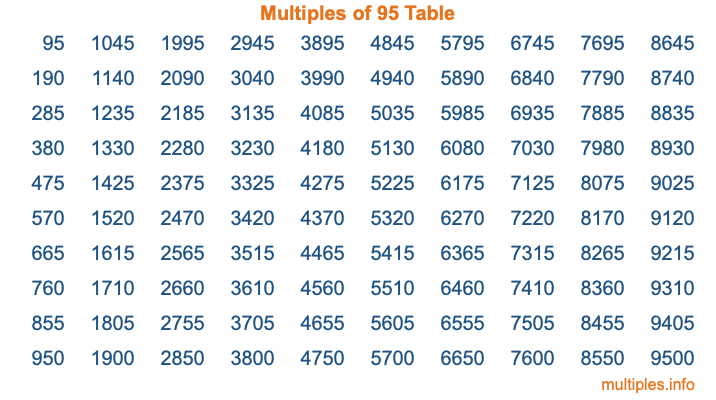Multiples of 95Welcome to the Multiples of 95 page. Here we will first teach you everything you will ever need to know about the multiples of 95, and then give you a study guide summary of everything we taught you to make sure you remember it all. Use this page to look up facts and learn information about the multiples of 95. This page will make you a multiples of ninety-five expert!

Definition of Multiples of 95
Multiples of 95 are all the numbers that when divided by 95 equal an integer. Each of the multiples of 95 are called a multiple. A multiple of 95 is created by multiplying 95 by an integer.

Therefore, to create a list of multiples of 95, you start with 1 multiplied by 95, then 2 multiplied by 95, then 3 multiplied by 95, and so on for as long as you want. Thus, the list of the first five multiples of 95 is 95, 190, 285, 380, and 475. To see a larger list of multiples of 95, see the printable image of Multiples of 95 further down on this page. We also have a category where you can choose any nth multiple of 95.

Multiples of 95 Checker
The Multiples of 95 Checker below checks to see if any number of your choice is a multiple of 95. In other words, it checks to see if there is any number (integer) that when multiplied by 95 will equal your number. To do that, we divide your number by 95. If the the quotient is an integer, then your number is a multiple of 95.

Is  a multiple of 95?

Least Common Multiple of 95 and ...
A Least Common Multiple (LCM) is the lowest multiple that two or more numbers have in common. This is also called the smallest common multiple or lowest common multiple and is useful to know when you are adding our subtracting fractions. Enter one or more numbers below (95 is already entered) to find the LCM.

Check out our LCM Calculator if you need more details about the Least Common Multiple or if you need the LCM for different numbers for adding and subtraction fractions.

nth Multiple of 95
As we stated above, 95 is the first multiple of 95, 190 is the second multiple of 95, 285 is the third multiple of 95, and so on. Enter a number below to find the nth multiple of 95.

th multiple of 95

Multiples of 95 vs Factors of 95
95 is a multiple of 95 and a factor of 95, but that is where the similarities end. All postive multiples of 95 are 95 or greater than 95. All positive factors of 95 are 95 or less than 95.

Below is the beginning list of multiples of 95 and the factors of 95 so you can compare:

Multiples of 95: 95, 190, 285, 380, 475, etc.

Factors of 95: 1, 5, 19, 95

As you can see, the multiples of 95 are all the numbers that you can divide by 95 to get a whole number. The factors of 95, on the other hand, are all the whole numbers that you can multiply by another whole number to get 95.

It's also interesting to note that if a number (x) is a factor of 95, then 95 will also be a multiple of that number (x).

Multiples of 95 vs Divisors of 95
The divisors of 95 are all the integers that 95 can be divided by evenly. Below is a list of the divisors of 95.

Divisors of 95: 1, 5, 19, 95

The interesting thing to note here is that if you take any multiple of 95 and divide it by a divisor of 95, you will see that the quotient is an integer.

Multiples of 95 Table
Below is an image of the first 100 multiples of 95 in a table. The table is in chronological order, column by column. The first column has the first ten multiples of 95, the second column has the next ten multiples of 95, and so on.The Multiples of 95 Table is also referred to as the 95 Times Table or Times Table of 95. You are welcome to print out our table for your studies.

Negative Multiples of 95
Although not often discussed or needed in math, it is worth mentioning that you can make a list of negative multiples of 95 by multiplying 95 by -1, then by -2, then by -3, and so on, to get the following list of negative multiples of 95:

-95, -190, -285, -380, -475, etc.

Multiples of 95 Summary
Below is a summary of important Multiples of 95 facts that we have discussed on this page. To retain the knowledge on this page, we recommend that you read through the summary and explain to yourself or a study partner why they hold true.

There are an infinite number of multiples of 95.

A multiple of 95 divided by 95 will equal a whole number.

95 divided by a factor of 95 equals a divisor of 95.

The nth multiple of 95 is n times 95.

The largest factor of 95 is equal to the first positive multiple of 95.

95 is a multiple of every factor of 95.

95 is a multiple of 95.

A multiple of 95 divided by a divisor of 95 equals an integer.

95 divided by a divisor of 95 equals a factor of 95.

Any integer times 95 will equal a multiple of 95.

Multiples of a Number
Here you can get the multiples of another number, all with the same attention to detail as we did for multiples of 95 on this page.

Multiples of
Multiples of 96
Did you find our page about multiples of ninety-five educational? Do you want more knowledge? Check out the multiples of the next number on our list!